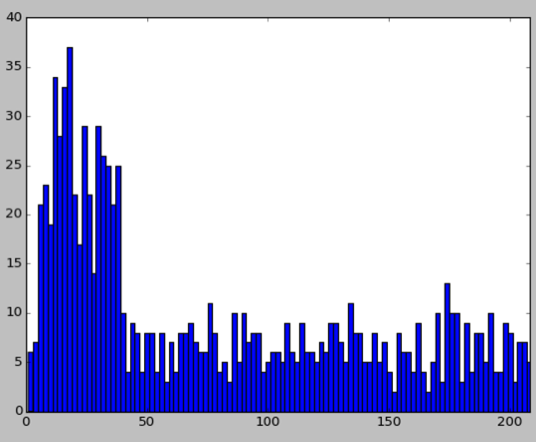Python: Creating a skewed random discrete distribution

I'm planning to write a variant of the TF/IDF algorithm over the HIMYM corpus which weights in favour of term that appear in a medium number of documents and as a prerequisite needed a function that when given a number of documents would return a weighting.

It should return a higher value when a term appears in a medium number of documents i.e. if I pass in 10 I should get back a higher value than 200 as a term that appears in 10 episodes is likely to be more interesting than one which appears in almost every episode.

I went through a few different scipy distributions but none of them did exactly what I want so I ended up writing a sampling function which chooses random numbers in a range with different probabilities:

``````
import math
import numpy as np

values = range(1, 209)
probs = [1.0 / 208] * 208

for idx, prob in enumerate(probs):
if idx > 3 and idx < 20:
probs[idx] = probs[idx] * (1 + math.log(idx + 1))
if idx > 20 and idx < 40:
probs[idx] = probs[idx] * (1 + math.log((40 - idx) + 1))

probs = [p / sum(probs) for p in probs]
sample =  np.random.choice(values, 1000, p=probs)

>>> print sample[:10]
[ 33   9  22 126  54   4  20  17  45  56]
``````

Now let's visualise the distribution of this sample by plotting a histogram:

``````
import matplotlib
matplotlib.use('TkAgg')
import matplotlib.pyplot as plt

binwidth = 2
plt.hist(sample, bins=np.arange(min(sample), max(sample) + binwidth, binwidth))
plt.xlim([0, max(sample)])
plt.show()
``````It's a bit hacky but it does seem to work in terms of weighting the values correctly. It would be nice if it I could smooth it off a bit better but I'm not sure how at the moment.

One final thing we can do is get the count of any one of the values by using the bincount function:

``````
>>> print np.bincount(sample)
4

>>> print np.bincount(sample)
16

>>> print np.bincount(sample)
3
``````

Now I need to plug this into the rest of my code and see if it actually works!